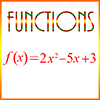# FunctionsThink of a function as a machine: You put a value in, and a value comes out. The function machine is also consistent. Thus, if you put in a specific value, like, say, 8, then a value comes out that depends only on 8. If your function machine produces 5 when you input 8, then it will always produce 5 when you input 8.

This page gives some simple examples of functions. The first example shows a function named f, which is defined by a quadratic polynomial. The second example show the composition of two functions, named f and g. And the third example talks about a sequence, which is a function whose domain is a set of integers.

Example 1)

If f(x) = 3 5x + 11, then what is f(5)?

A. −47
B. −43
C. – 39
D. −35
E. −33

Explanation: To solve this problem, we’ll substitute -5 for x:

f(x) = -3x² – 5x + 11
f(-5) = -3(-5)² – 5(-5) + 11
= (-3 × 25) + 25 + 11
= -75 + 25 + 11
= -39

Example 2)

If f(x) = -3x – 9 and g(x) = 3x + 23, then what is f(g(-9))?

A. 2
B. 3
C. 4
D. 5
E. 6

Explanation: We’ll start with the inside part of the question and work our way out. We need to first determine what g(-9) is, so we’ll substitute the -9 for x in the g(x) function.

g(x) = 3x + 23
g(-9) = 3(-9) + 23
= -27 + 23
= -4

We’ll now substitute the -4 for x in the f(x) function.

f(x) = -3x – 9
f(-4) = -3(-4) – 9
= 12 – 9
= 3

So B is the correct answer.

Example 3)

If a sequence starts at 17, and ends at -4, with each number being 3 less than the previous number, how many numbers are in the sequence?

Explanation: The difference between the first term, 17, and the last term, – 4, is:

17 – (-4) = 21

And since each term is 3 different from the previous term, then there must be 21/3 = 7 terms from 17 to -4.

But that doesn’t include the first number in the sequence, 17. So there are actually 7 + 1 = 8 numbers in the sequence.